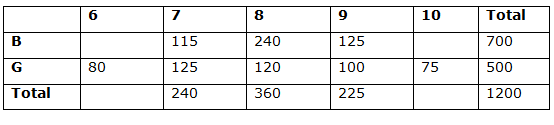# SBI Clerk Pre Quantitative Aptitude (Day-27)

Dear Aspirants, Our IBPS Guide team is providing new series of Quantitative Aptitude Questions for SBI Clerk Prelims 2020 so the aspirants can practice it on a daily basis. These questions are framed by our skilled experts after understanding your needs thoroughly. Aspirants can practice these new series questions daily to familiarize with the exact exam pattern and make your preparation effective.

Start Quiz

Ensure Your Ability Before the Exam – Take SBI Clerk 2020 Prelims Free Mock Test

Data Interpretation

Directions (1 – 5): Study the following information carefully and answer the questions given below?

Pie graphs show the % of applications received from various states in 2017 and 2018

1) What is the difference between the no. of applications received from A and C in 2017 and B, C and F in 2018?

a) 1568

b) 1398

c) 2076

d) 1386

e) None of these

2) If 36% of total no. of applications are rejected in 2017 and no. of applications rejected from D is 194, what is the approximate% of valid applications from D to total no. of applications?

a) 84%

b) 65.44%

c) 52.02%

d) 79.54%

e) None of these

3) What is the ratio of the no. of applications from A and E in 2017 to A, B, D in 2018 if 420 applicants apply from state A instead of E both the years?

a) 84:123

b) 64:161

c) 75:81

d) 72:121

e) None of these

4) If 20% of applicants are male in state D in 2017 and 40% of applicants are female in state C in 2017. The no. of female applicants from D is how much more/less than the no. of male applicants from C?

a) 3278

b) 3192

c) 2059

d) 2166

e) None of these

5) In 2018, 25% of applicants from state D and 35% of applicants from state E apply from state A, the no. of applicants from state A?

a) 7684

b) 6554

c) 6678

d) 7379

e) None of these

Caselet

Directions (6 – 10): Study the following information carefully and answer the questions given below?

A total of 1200 students are studying in a school. There are 5 classes in the school from 6 to 10. The ratio of the number of boys to girls is 7: 5. In class 8, 2/3rd students are boys. 20% of students belong to class 7. 80 is the number of girls students in class 6. A total of 360 students are in class 8. Number of boys in class 9 is 125% of girls in that class where the number of girls in class 9 is 20% of total number of girls. Class 10 has 75 girls. The ratio of the number of boys to girls in class 7 is 23:25

6) What is the ratio between the no. of boys in 8th and the no. of girls in 7th?

a) 48:25

b) 45: 28

c) 24: 35

d) 28: 37

e) None of these

7) If the total no. of students in class 6 is 200, the no. of boys from 6, 7, 8 together form what % of total no. of boys?

a) 48.55%

b) 45.78%

c) 67.85%

d) 57.76%

e) None of these

8) What is the difference between the average of boys from 7, 8, 9 together and the average of girls from 6, 7, 8 together?

a) 48.25

b) 51.66

c) 24.35

d) 58.97

e) None of these

9) If the no. of boys in class 10 is 100, the difference between class 10 boys and class 9 boys are how much less than the difference between class 8 girls and class 6 girls?

a) 15

b) 40

c) 25

d) 45

e) None of these

10) What is the total number of students in classes 7, 8, 9?

a) 925

b) 540

c) 746

d) 825

e) None of these

Directions (1-5) :

A&C in 2017 = 35% 11400 = 3990

B, C, F in 2018 = 32% 16800 = 5376

Diff = 1386

36% of 11400 = 4104

No. of valid app = 11400 – 4104 = 7296

Total no. of app from D = 35% of 11400 = 3990

Total no. of valid app from D = 3990 – 194 = 3796

3796 / 7296 * 100 = 52.02%

30% of 11400 = 3420

3420 + 420 = 3840 – – – 1

55% of 16800 = 9240

9240 + 420 = 9660 – – – 2

Now, 3840: 9660 = 64: 161

80% of 35% of 11400 – 60% of 15% of 11400

3192 – 1026 = 2166

25% of 16% of 16800 + 35% of 25% of 16800 + 27% of 16800

672 + 1470 + 4536 = 6678

Directions  (6 – 10):Required ratio

= > 240 : 125 = 48:25

6th boys = 200 – 80 = 120

6, 7, 8 boys = 475

Required percentage = 475 / 700 * 100 = 67.85%

Required difference

= > 480 / 3 – 325 / 3 = 155 / 3 = 51.66

Required difference

= > (125 – 100) – (120 – 80) = 15